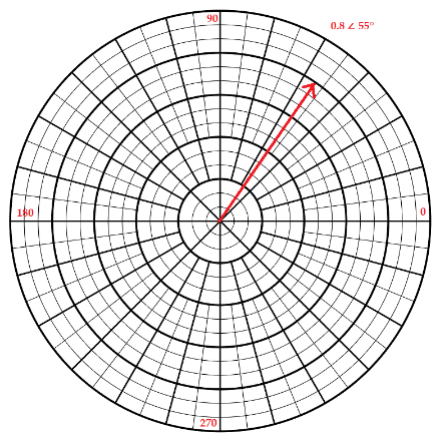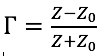|
|### What is a Reflection Coefficient?

March 22, 2023

Just as a beam of light might bounce off a sheet of glass, some or all of an RF signal may reflect from an impedance other than one equal to the characteristic impedance of the transmission line. If the characteristic impedance is Z0 and the encountered impedance is Z, then:If the encountered impedance is complex, then the reflection coefficient is also complex. If Z = Z0, then Γ = 0 and there is no reflection. If Z is 0, a short, then Γ = -1 and the reflection is total but inverted. If Z is infinite, an open, then Γ = 1 and again the reflection is total but in phase.

For complex impedances, the reflection coefficient will also be complex, and may be expressed as a vector with magnitude less than one and some angle such as 0.5∠53°. It can be expressed in Real/Imaginary Cartesian coordinates as well, such as 0.3+j0.4 which is the same vector. Figure 1 depicts a reflection coefficient as a vector on a polar chart.Figure 11 – Vector on a Polar Chart

See this and more important radio frequency charts and formulas in this comprehensive application note